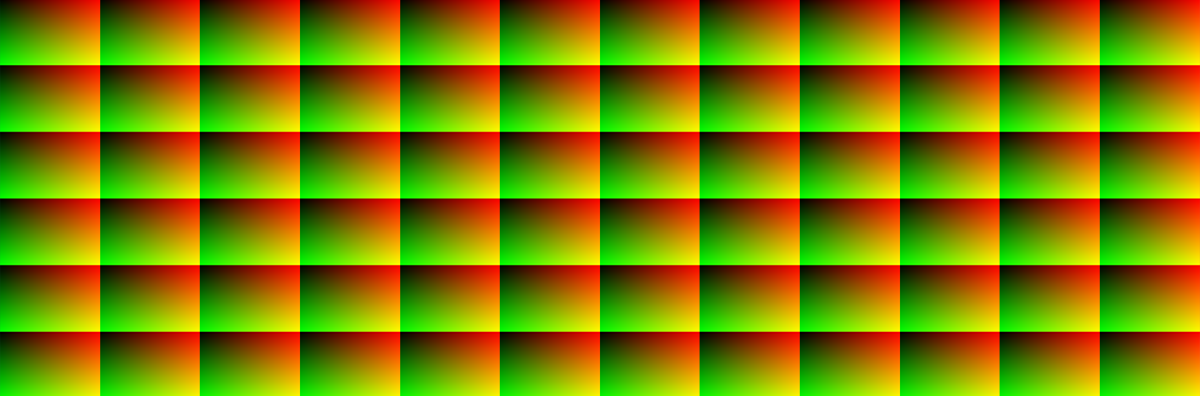# GridsMaking grids is very useful if you want to, for example, repeat an operation in different places of the screen. The key component to make a grid is the `fract()` function. It takes in a float value and returns only the fractional part of it. So, 3.14159 would return 14159.

If you display the fractional part of the UV multiplied with some value the screen will be chopped up into that amount of sections – just like a grid – with each cell having values from 0 to 1.

``````vec2 grid(vec2 uv, float columns, float rows){
return fract(vec2(uv.x * columns, uv.y * rows));
}

void fragment() {
vec2 grid = grid(UV, 5.0, 6.0);
COLOR = vec3(grid.r, grid.g, 0.0);
}
``````

To keep track of which grid cell the current pixel is in you can use the `floor()` function. Instead of returning the fractional part, it returns the integer part. So, 3.14159 would return 3. Use it in the same way as `fract()` and you will get an index of each cell, which you then can use, for example, in a loop.

``````vec2 grid = fract(UV * 5.0);
vec2 cell_index = floor(UV * 5.0) / 5.0;
``````

See this eposode of The Art of Code for a cool use of grids.